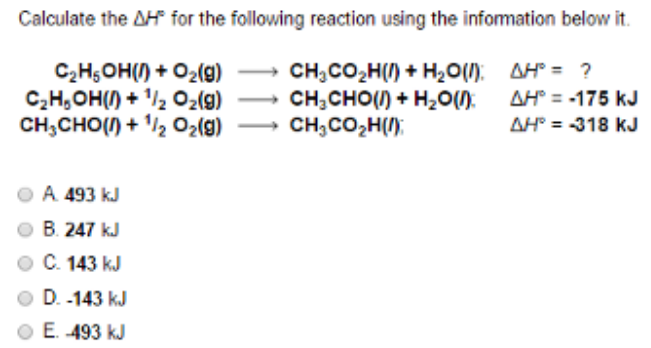# Problem: Calculate the ΔH° for the following reaction using the information below it. C2H5OH (l) + O2 (g) → CH3CO2H (l) + H2O (l): ΔH° = ? C2H5OH (l) + 1/2O2 (g) → CH3CHO(l) + H2O(l): ΔH° = -175 kJ CH3CHO (l) + 1/2O2 (g) → CH3CO2H (l): ΔH° = -318 kJ (A) 493 kJ (B) 247 kJ (C) 143 kJ (D) -143 kJ (E) -493 kJ

🤓 Based on our data, we think this question is relevant for Professor Zellmer's class at OSU.

###### FREE Expert Solution###### Problem Details

Calculate the ΔH° for the following reaction using the information below it.

C2H5OH (l) + O2 (g) → CH3CO2H (l) + H2O (l): ΔH° = ?

C2H5OH (l) + 1/2O2 (g) → CH3CHO(l) + H2O(l): ΔH° = -175 kJ

CH3CHO (l) + 1/2O2 (g) → CH3CO2H (l): ΔH° = -318 kJ

(A) 493 kJ

(B) 247 kJ

(C) 143 kJ

(D) -143 kJ

(E) -493 kJ